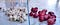# Imbalanced data and Linear regression“Necessity is the mother of invention”

Linear Regression (LR) is used for finding linear relationship between target and one or more predictors. The core idea is to obtain a line that best fits the data. The best fit line is the one for which total prediction error(all data points) are as small as possible. Error is the distance between the point to the regression line. In this blog we will cover various set hyper parameter of linear regression model and performing prediction models.

Linear regression assumes that the relationship between the features and the target vector is approximately linear. That is, the effect (also called coefficient…## koshal singh

MSc. Big Data Analytics student @ central university of rajasthan. #mlispassion